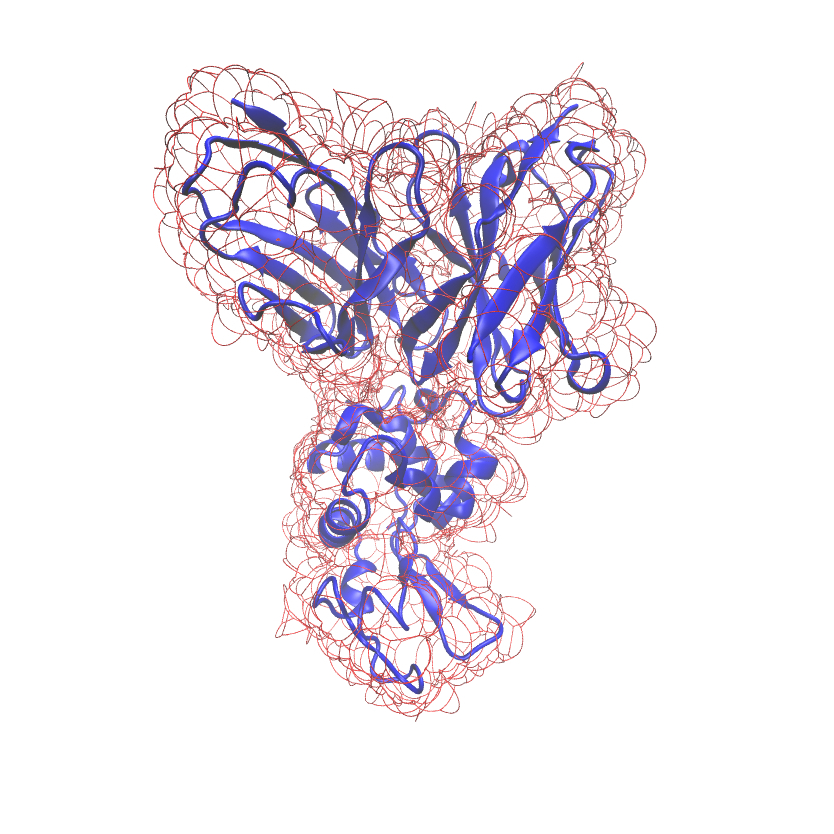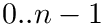Structural Bioinformatics Library Template C++ / Python API for developping structural bioinformatics applications.
User Manual# Union_of_balls_boundary_3

Authors: F. Cazals and T. Dreyfus

# Introduction

This package provides a generic geometric data structure and the accompanying algorithm to compute the boundary of an union of a family of 3D balls. The boundary is represented using a combinatorial data structure that is warranted to be exact. Two options are provided to handle the construction intersection points (found at the intersection of three spheres); their coordinates can indeed be represented exactly (using degree two algebraic numbers), or using interval arithmetics.

# Algorithms

## Representation

The boundary of an union of 3D balls is represented with a data structure composed of three items :

• the vertices representing the intersection points of three balls,
• the arcs representing circle arc at the intersection between two balls,
• the spherical caps representing spherical polygons defining the boundary of the union.

The specification of the boundary involves two types of information:

• the combinatorial information : how vertices, arcs and spherical are connected; this information can be computed exactly.
• the geometric information : the embedding i.e. the coordinates of the vertices. These coordinates involve the roots of degree two polynomials defined from the specification (centers, radii) of the input balls (center, radii).

Hence, our representation of the boundary decouples these two aspects, based on the half-edge data structure from the CGAL library . This structure is composed of :

• faces : they represent the spherical caps;
• half-edges : they represent the oriented arcs bounding the spherical caps; more precisely, for two adjacent faces sharing an arc, the arc is represented by two half-edges with opposite directions;
• vertices : they represent the intersection points.

We now describe the different steps to build the boundary with this data structure.

## Steps

Consider the-complex of an input family of 3D balls : it is a filtration of the simplices (vertices, edges ,triangles and tetrahedra) of an underlying Delaunay/regular triangulation. Each simplex has a status :

• singular when the simplex has no coface in the-complex,
• interior when all its cofaces are in the-complex,
• regular otherwise.

Also, by construction, it is possible to create an artificial vertex located at the infinite, so that it is easy to find the simplices corresponding to the boundary of the union of balls. In particular, a tetrahedron containing an infinite vertex is called exterior and allows to define which part of the boundaries are exterior or bound cavities.

The overall algorithm computing the boundary of the union uses the-complex , and goes through six steps:

1. building the vertices: this is done by visiting the triangles in the-complex. When the triangle is interior, none of the two intersection points of the three 3D balls represented by the triangle are at the boundary. When regular, there is exactly one intersection point at the boundary, and when singular, there are exactly two intersection points. Hence, we can totally determine the set of vertices.

2. building the half-edges: this is done by visiting the edges in the-complex. When the edge is interior, there is no intersection arc on the boundary. When singular, the intersection arc is a full circle and we just add a pair of opposite half-edges. When regular, there are possibly multiple intersection arcs, and we need to visit each vertex bounding the arcs to reconstruct a pair of opposite half-edges for each arc. To know which pair of vertices are the end-points of the same arc, we simply visit the incident pairs of triangles in the-complex bounding a same tetrahedron that is not in the-complex.

3. linking the half-edges: this is done by grouping all half-edges corresponding to intersection arcs at the surface of a ball, then by linking pairs of half-edges for which the end-point of the first one is the start-point of the second one. Since half-edges are paired and are oriented, we can always associate to half-edges the bounding ball on their left, allowing to easily group them. Then, a Union-Find algorithm over the vertices bounding the half-edges in the same group allows to link those half-edges.

4. building the faces: this is done by visiting the vertices in the-complex. An interior vertex has no associated spherical cap on the boundary. A singular vertex corresponds to a full sphere, represented by a unique face with no bounding half-edge. When regular, we need to know how many not connected spherical caps are bounding the corresponding 3D ball : this is done by grouping adjacent tetrahedra of the underlaying triangulation that are not in the-complex. A simple Union-Find algorithm allows to group those adjacent tetrahedra, defining one face per independent set; For each face, we also need to determine the boundaries of this face. To do so, we use again a Union-Find algorithm for grouping the connected half-edges around a face. Each connected component of half-edges is called a CCB. We arbitrarily set the first CCB of each face as its face boundary, all other ones defining its face holes.

5. linking the faces: this is done by simply running an Union-Find algorithm over the half-edges for linking all the adjacent faces. At the end of the process, the resulting connected components are the different connected components of the boundary of the union of input 3D balls.

6. finding the exterior and cavity boundaries: this is done by simply running an Union-Find algorithm over all tetrahedra of the underlaying triangulation that are not in the-complex : the connected component having infinite tetrahedra correspond to exterior connected components of the boundary, while all other ones correspond to cavities' boundary connected components.

# Implementation

## Data Structure

The boundary is represented by the class SBL::GT::T_Union_of_balls_boundary_3_data_structure< WeightedAlphaComplex3 , IS_CCW , HalfedgeDSBase >. This data structure needs a builder providing an algorithm to build it, either from a family of 3D balls, either directly from an input-complex. The underlying data structure is an half-edge data structure. Such a structure is made of vertices, oriented edges and faces : each adjacent faces are connected through a pair of opposite oriented edges, hence the name half-edges. In this case, the half-edges represent the intersection arcs / circles between the surface of the 3D balls, thus defining the faces as the connected pieces of surfaces for each sphere, and the vertices as the intersection points between the arvs. The orientation of all half-edges around their bounding face is set to be the same for all faces, that is either clockwise(CW) or counter-clockwise (CCW). (As detailed below this is set by a tag.)

The three template parameters are :

The main functionality provided by this data structure are :

• traversing : in addition of the base way to explore the data structure (iterating over vertices, half-edges, and faces, or iterating on successive half-edges), it is possible to iterate over the set of holes of a face, (SBL::GT::T_Union_of_balls_boundary_3_data_structure::holes_begin, SBL::GT::T_Union_of_balls_boundary_3_data_structure::holes_end) and over the connected components of faces of the boundary (SBL::GT::T_Union_of_balls_boundary_3_data_structure::connected_components_of_faces_begin, SBL::GT::T_Union_of_balls_boundary_3_data_structure::connected_components_of_faces_end)

• accessing : it is possible to access geometric information such as the geometric point corresponding to a vertex (SBL::GT::T_Union_of_balls_boundary_3_data_structure::get_point), the geometric arc (SBL::GT::T_Union_of_balls_boundary_3_data_structure::get_circular_arc) or supporting circle (SBL::GT::T_Union_of_balls_boundary_3_data_structure::get_supporting_circle) corresponding to half-edge, and the geometric supporting sphere (SBL::GT::T_Union_of_balls_boundary_3_data_structure::get_supporting_sphere) corresponding to a face; note that the boundary data structure is combinatorial, meaning that these geometric constructions require an appropriate geometric kernel to be built; thus, the corresponding methods are templated by a spherical kernel as defined in the CGAL 3D Spherical Kernel user manual

## Builder

The algorithm described in section Algorithms is implemented in the builder class

SBL::GT::T_Union_of_balls_boundary_3_builder< UnionOfBallsBoundaryDS , ExactNT >. It takes as input a range of 3D balls, or an already computed-complex, and computes the associated boundary. Note that the algorithm is parametrized by a dimension parameter specifying if only vertices should be built, or vertices and half-edges, or vertices, half-edges and facets.

The two template parameters are :

• UnionOfBallsBoundaryDS : representation of the boundary of the union of 3D balls, such as SBL::GT::T_Union_of_balls_boundary_3_data_structure;

• ExactNT : representation of an exact number type, like rational numbers; it is by default the rational number representation from CGAL : Gmpq

## Module

This package also provides the module SBL::Modules::T_Union_of_balls_boundary_3_module< ModuleTraits , ExactNT > that computes the boundary of the union of a family of 3D balls that are represented by a-complex data structure :

The two template parameters are:

• ModuleTraits : traits class of the module defining the type Weighted_alpha_complex_3 and Union_of_balls_boundary_3,
• ExactFT : the exact number type used within the builder SBL::CSB::T_Union_of_balls_boundary_3_builder, as specified in section Builder .

An example of usage of this module is shown in section Using the boundary module .

# Examples

## Building the boundary of an union of balls

This example shows how to simply compute the boundary of an input union of 3D balls and print some statistics about this boundary.

## Using the boundary module

This example shows how to instantiate the provided module SBL::Modules::T_Union_of_balls_boundary_3 in a biological context by computing the boundary of a molecule loaded from a PDB file:

## Printing a VMD output

This example shows how to print a VMD output representing the boundary of an input family of 3D balls :